# Question 5# Question 7

• MC = W/MPL# Question 8

• Operate if Loss < Fixed Cost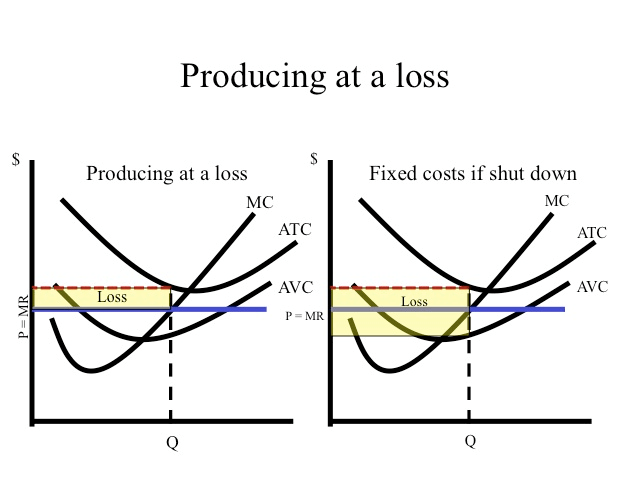• As demonstrated in the table, if the price is below average total cost but above average variable cost, losses are minimized by producing 50 units and incurring a loss of \$3.50.

• If the firm shut down, it would still have to pay fixed cost of five.

• Since average variable costs at 50 units is 42 cents and the price is 45 cents, it covers the variable costs and contributes three cents on each unit toward the paying the fixed costs.

• Three cents times 50 units is \$1.50 which is the amount the firm has reduced their loss by producing instead of shutting down.

# Question 9

• In the long-run the profit for a monopolistically competitive frim is 0# Question 11

• The Marginal Revenue of a firm in a perfectly competitive industry is constant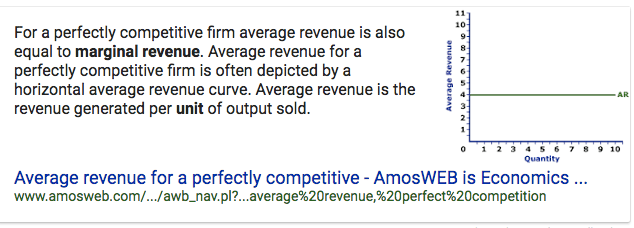# Question 12

• Cost Minimization

• Optimal Input Mix is where MPL/Wage = MPK/Rental Rate

• If MPL/Wage > MPK/Rental Rate, then hire the human worker

• If MPK/Rental Rate > MPL/Wage, then the machines win! use more capital

# Question 15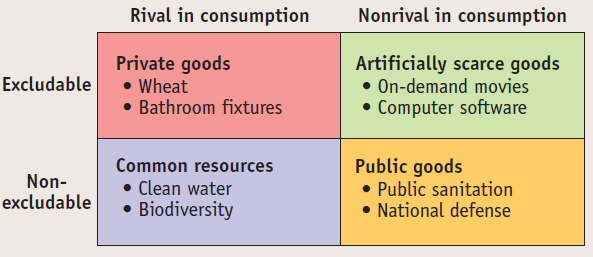# Question 18

• Price elasticity and tax share# Question 19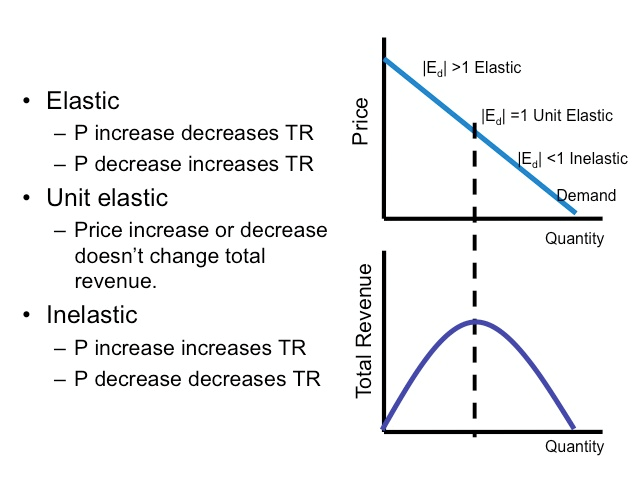# Question 24

• P = MC --> Must be Perfectly Competitive# Question 26

• The Effect of an Increase in Demand

• An increase in the demand for a product causes the equilibrium price and quantity to increase in the market.

• An increase in demand raises price and profit, which causes more suppliers to enter the market

• Higher industry output from new entrants drives price and profit back down to its original equilibrium# Question 28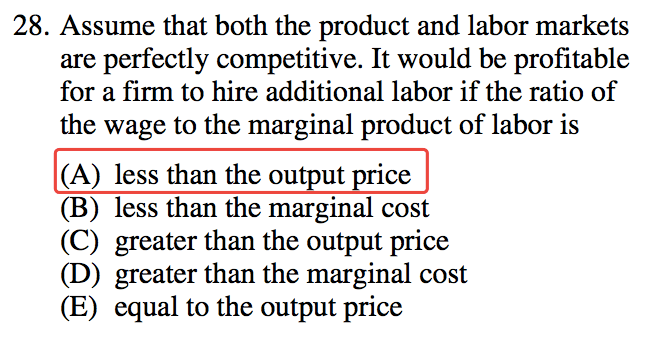# Question 37

• For a monopolistically competitive profit-maximizing firm, AR = P# Question 38

• The short-run supply curve for a firm in a perfectly competitive industry is its marginal cost curve above the minimum point of its average variable cost curve

• In the short run the firm needs only to cover its variable costs (at Q1 below) – this is largely because covering variable cost ensures than an output can be produced in the future - if variable costs cannot be covered then no further output can be made.

• In addition, fixed costs have already been paid for prior to any marginal decision to supply, so do not enter into the firm’s short run calculations.# Question 44# Question 49

![[rnp3Ct ofa fill in the price of good X Nanue o f Good X Normal Good inferior Good Giffen Good Substitution t ion of Change) in quantity demanded of Good X Increase in quantity demanded of Good X in quantity demanded of Good X Inco me E # s titufrn & Imome (Direc lion of Change) (Bdagritude of Change) Inciease in quantity demanded o? Good X Decrease in guaMity demanded of Good X Decrease in quartity demanded of Good X SE Price P ogitive F o gifve Ne ga tivs ](./media/image300.png)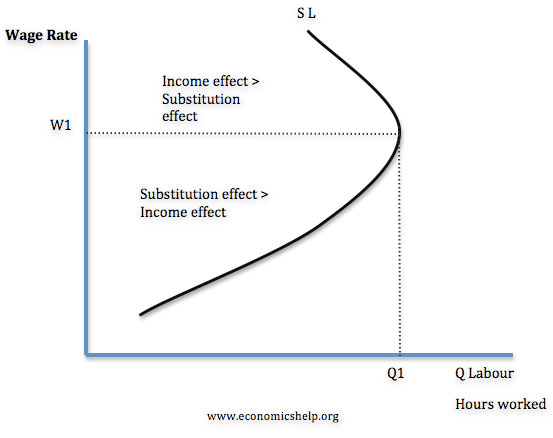# Question 51

• A profit-maximizing monopolist selects its output level in the elastic region of its demand curve.# Question 56